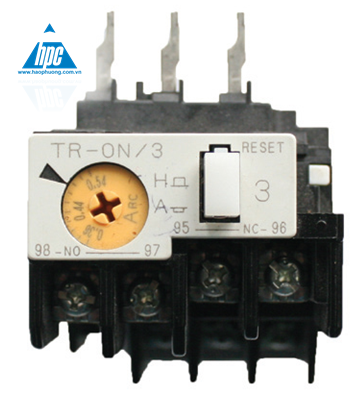# Instruction on how to select the thermal relay### When designing electric motor cabinets

Thermal relays used for thermal overload protection are indispensable. However, when choosing, people still wonder how to choose the best to ensure the engine is working in the best way and cutting load when the heat is.For thermal relays as well as contactors, we must calculate the rated working current of the motor.

### When selecting the thermal relay, the main concern is the following Specifications:

– Rated current
– Which Product line is the most suitable for contactor (each type of thermal relay is compatible with a corresponding contactor, the manufacturer has recommended choosing the right one on the product catalog)

-Similar to calculating the current for selecting contactor, we calculate the rated current, then select the following.

– Idm = Itt x 2
– Iccb = Idm x 2
– Ict = (1,2-1,5)Idm

#### We calculate in a specific example as follows:

With 3-phase motor, 380V, 3kW, calculate the rated current according to the following formula:
Itt = P/(1.73 x 380 x 0,85)here, the power factor is 0,85.
then we have Itt = 3000/(1,73x380x0,85)=5,4A

The thermal relay line we select with a start factor of 1.2-1.4 times Idm, we choose the thermal relay line as:
Idm = 1,4xItt = 1,4×5,4=7,6A.

So the line of the thermal relay is 8A. Thermal relays often have a range of current correction, working current set, we can choose the residual current range to be adjustable when using real load.